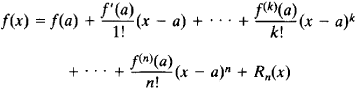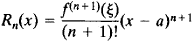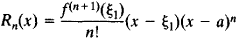# Taylor's Formula

The following article is from The Great Soviet Encyclopedia (1979). It might be outdated or ideologically biased.

## Taylor’s Formula

the formulaIf f is a function having an nth derivative at the point x = a, then Taylor’s formula provides a representation of f as the sum of a polynomial in (xa) of degree n and a remainder Rx(x). In the neighborhood of a, Rn (x) is an infinitesimal of higher order than (xa)n—that is, Rn(x) = αn(x) (xa)n, where αn(x) → 0 as xa.

If the (n + 1)th derivative exists on the interval between a and x, then the remainder may be expressed in Lagrange’s form or in Cauchy’s form. Lagrange’s form isCauchy’s form isHere, ξ, and ξ1 are some points on the given interval.

At the point a, the order of contact of the graph of the polynomial in Taylor’s formula and the graph of f(x) is at least n.

Taylor’s formula is used in the study of functions and in approximate calculations.

### REFERENCES

Khinchin, A. Ia. Kratkii kurs matematicheskogo analiza. Moscow, 1953.
Fikhtengol’ts, G. M. Kurs differentsial’nogo i integral’nogo ischisleniia, 7th ed., vol. 1. Moscow, 1969.
Mentioned in ?
References in periodicals archive ?
Odibat, "A novel method for nonlinear fractional partial differential equations: combination of DTM and generalized Taylor's formula," Journal of Computational and Applied Mathematics, vol.
Shawagfeh, "Generalized Taylor's formula," Applied Mathematics and Computation, vol.
This framework will be illustrated by a simple combinatorial proof and interpretation of Taylor's formula in Section 2.1 and equations (5) and (6) in Sections 2.2 and 3.
Thirdly, Bernanke criticized the Taylor's formula that it reacts to short-term movements of inflation.
By Taylor's formula hence [MATHEMATICAL EXPRESSION NOT REPRODUCIBLE IN ASCII.]
We may observe here that if f(x) = [x.sup.q], x [member of] [R.sub.0], q [member of] [N.sub.0], then by Taylor's formula it follows that
Keywords and Phrases: Ostrowski and Gruss type inequalities, Differentiable functions, Estimates, Taylor's formula, Lagrange form of reminder.
Taylor's formula makes quite good sense when comparing apples to apples; that is, .444 Marlin to .45-70 to .50 Alaskan.
Taylor's formula is simple, tried and true--biographical musicals based on the lives of major black entertainment figures.
By introducing an analogue of Taylor's formula for [x.sup.n], n [member of] Z, we can show the local, quadratic convergence independently of the general theory.

Site: Follow: Share:
Open / Close